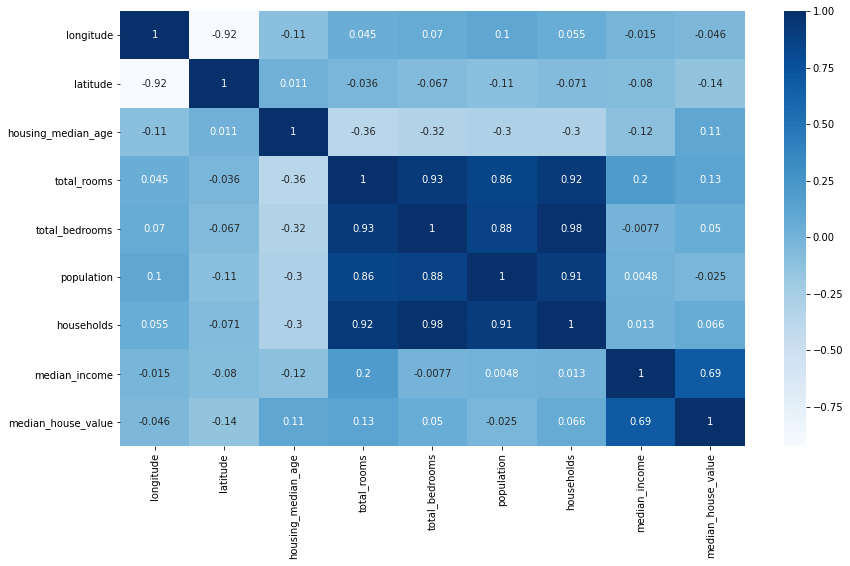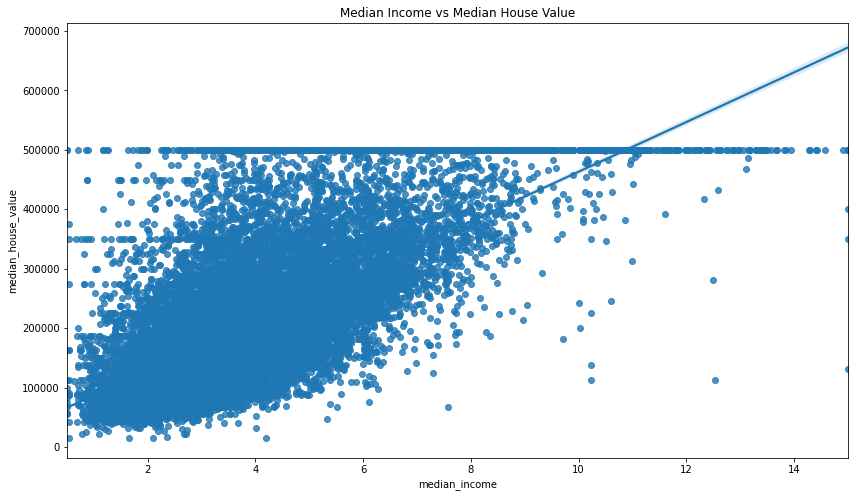Picture by Lumn, Pexels.

Spearman’s rank correlation coefficient, sometimes called Spearman’s rho, is a nonparametric statistic used to measure rank correlation, or the statistical dependence between the rankings of two variables. It explains how well a statistical relationship between two variables can be explained using a monotonic function.

While Pearson’s correlation coefficient is used to measure the relationship between pairs of variables with a linear relationship, Spearman’s rank correlation coefficient assesses monotonic relationships, whether they’re linear or not. It’s a non-parametric measure of correlation, meaning that it doesn’t assume that the data is normally distributed.

As the name suggests, it’s a measure of rank correlation, meaning that it’s based on the ranks of the data rather than the data itself. This is important because it means that it’s not affected by outliers, which can skew Pearson’s correlation coefficient. Spearman’s rank correlation is equal to the Pearson correlation coefficient of the ranks of the data and, like Pearson’s correlation coefficient, it ranges from -1 to 1.

### When should you use Spearman’s rank correlation coefficient?

Spearman’s rank correlation coefficient is a nonparametric measure of the monotonicity of the relationship between two datasets. Unlike the Pearson correlation, the Spearman correlation does not assume that both datasets are normally distributed.

It is often used as an alternative to the Pearson correlation in the presence of outliers and can be useful for both continuous and ordinal variables. It assumes that the data must at least be ordinal and the scores on one variable need to be related monotonically to the other variable in the pairwise comparison.

It’s typically used on ordinal or continuous variables, but it can also be used on nominal variables. It’s often used in place of Pearson’s correlation coefficient when the data is not normally distributed.

### Spearman’s rank correlation coefficient using corr()

The Pandas `corr()` function can be used to compute pairwise correlation coefficients, including Spearman’s rho, on Pandas dataframe values, and can either calculate them as individual pairs (i.e. top speed and price), or as pairs across an entire dataframe.

By default, the `corr()` function calculates the more commonly used Pearson’s correlation coefficient, but it can be easily modified to return the Spearman’s rank correlation coefficient, or Spearman’s rho, by passing in the optional argument `method='spearman'`. Here’s a quick summary of the parameters you can pass to the Pandas `corr()` function.

Parameter Description
`method` The `method` parameter defines which of the three correlation coefficient methods to use. The default is `pearson`, which is the Pearson product-moment correlation coefficient. The other two methods are `kendall`, for the Kendall Tau correlation coefficient, and `spearman` for the Spearman rank correlation coefficient.
`min_periods` The `min_periods` parameter defines the minimum number of observations required to calculate the correlation coefficient. If there are fewer than `min_periods` observations, the correlation coefficient will be set to `NaN`. This currently only works when the `method` parameter is set to `pearson` or `spearman`.
`numeric_only` The `numeric_only` parameter was new in Pandas 1.5.0, so it won't work on earlier releases of Pandas. This parameter defines whether to only calculate the correlation coefficient on numeric columns. If set to `True`, only numeric columns will be used to calculate the correlation coefficient. If set to `False`, all columns will be used to calculate the correlation coefficient. The default is `True`. In a future release, the value of `numeric_only` will change to `False`, so if you don't set it you'll currently get this warning: "FutureWarning: The default value of numeric_only in DataFrame.corr is deprecated. In a future version, it will default to False. Select only valid columns or specify the value of numeric_only to silence this warning." Passing `numeric_only=True` will fix it.

#### Interpreting Spearman’s rho

When you use the optional `method='spearman'` option, the `corr()` function will return the Spearman’s rank correlation coefficient for each pair of columns in the dataframe. The correlation coefficient returned, will be a value between -1 and +1. Here’s how you can interpret what these coefficients mean:

Spearman's rho value Strength of relationship
-1 Perfect negative linear relationship
-0.7 Strong negative linear relationship
-0.5 Moderate negative linear relationship
-0.3 Weak negative linear relationship
0 No linear relationship
0.3 Weak positive linear relationship
0.5 Moderate positive linear relationship
0.7 Strong positive linear relationship
1 Perfect positive linear relationship

To work through some simple examples showing how to compute Spearman’s rank correlation in Python we’ll be using Pandas. To get started, import Pandas in a Jupyter notebook. We’ll also use the Matplotlib and Seaborn data visualisation libraries to visualise the Spearman rank correlation, and we’ll use Scipy Stats to test the statistical significance.

``````import pandas as pd
import matplotlib.pyplot as plt
import seaborn as sns
from scipy.stats import spearmanr
``````

### Import a dataset

Next, import a dataset into Pandas that contains data with linear relationships. I’m using a house price dataset.

``````df = pd.read_csv('https://raw.githubusercontent.com/flyandlure/datasets/master/housing.csv')
``````
0 1 2 3 4
longitude -122.23 -122.22 -122.24 -122.25 -122.25
latitude 37.88 37.86 37.85 37.85 37.85
housing_median_age 41.0 21.0 52.0 52.0 52.0
total_rooms 880.0 7099.0 1467.0 1274.0 1627.0
total_bedrooms 129.0 1106.0 190.0 235.0 280.0
population 322.0 2401.0 496.0 558.0 565.0
households 126.0 1138.0 177.0 219.0 259.0
median_income 8.3252 8.3014 7.2574 5.6431 3.8462
median_house_value 452600.0 358500.0 352100.0 341300.0 342200.0
ocean_proximity NEAR BAY NEAR BAY NEAR BAY NEAR BAY NEAR BAY

### Calculate the Spearman’s rank correlation for a pair of columns

To calculate Spearman’s rank correlation on a single pair of Pandas dataframe columns we’ll use the Pandas `corr()` function with the `method` parameter set to `spearman`. To use the function we run `df['median_income'].corr(df['median_house_value'], method='spearman')`, where the first column is the column we want to compare to the second column.

``````df['median_income'].corr(df['median_house_value'], method='spearman')
``````
``````0.6767781095942506
``````

### Calculate the Spearman’s rank correlation for all columns in the dataframe

To calculate the Spearman’s rank correlation for all columns in a Pandas dataframe we can use the `corr()` method with the `method` parameter set to `spearman`, but we’ll assign this to `df` rather than a single column, and we won’t pass in another column to compare against.

At present, you’ll need to set `numeric_only=True` to avoid getting a FutureWarning about the default value changing to `False` in a future version of Pandas.

``````df.corr(numeric_only=True, method='spearman')
``````
longitude latitude housing_median_age total_rooms total_bedrooms population households median_income median_house_value
longitude 1.000000 -0.879203 -0.150752 0.040120 0.063879 0.123527 0.060020 -0.009928 -0.069667
latitude -0.879203 1.000000 0.032440 -0.018435 -0.056636 -0.123626 -0.074299 -0.088029 -0.165739
housing_median_age -0.150752 0.032440 1.000000 -0.357162 -0.306544 -0.283879 -0.281989 -0.147308 0.074855
total_rooms 0.040120 -0.018435 -0.357162 1.000000 0.915021 0.816185 0.906734 0.271321 0.205952
total_bedrooms 0.063879 -0.056636 -0.306544 0.915021 1.000000 0.870937 0.975627 -0.006196 0.086259
population 0.123527 -0.123626 -0.283879 0.816185 0.870937 1.000000 0.903872 0.006268 0.003839
households 0.060020 -0.074299 -0.281989 0.906734 0.975627 0.903872 1.000000 0.030305 0.112737
median_income -0.009928 -0.088029 -0.147308 0.271321 -0.006196 0.006268 0.030305 1.000000 0.676778
median_house_value -0.069667 -0.165739 0.074855 0.205952 0.086259 0.003839 0.112737 0.676778 1.000000

### Visualise Spearman’s rank correlation coefficients with a heatmap

By using Seaborn, we can visualise the Spearman’s rank correlation coefficients using a heatmap visualisation of correlation coefficients. This can be an effective way to quickly understand relationships between data, as you can simply lookup those values where the correlation is strongly negative or strongly positive.

``````plt.figure(figsize=(14,8))
sns.heatmap(df.corr(numeric_only=True), annot=True, cmap='Blues')
``````
``````<AxesSubplot:>
``````### Visualising correlations between pairs of columns

You can also use Seaborn to examine the relationship between a specific pair of variables that appear to have a strong correlation. The Seaborn `regplot()` function is great for this.

``````plt.figure(figsize=(14,8))
sns.regplot(x='median_income', y='median_house_value', data=df)
plt.title('Median Income vs Median House Value')
``````
``````Text(0.5, 1.0, 'Median Income vs Median House Value')
``````### Calculate the statistical significance of the correlation coefficient

Finally, you can measure the statistical significance of Spearman’s rho using Scipy Stats. Scipy Stats calculates both the correlation coefficient of a pair of variables as well as their statistical significance, but it’s clunkier to use than Pandas, so tends to be used mostly to validate whether a correlation is significant or not.

To use it, you simply call `spearmanr()` and pass in the two Pandas columns you want to compare. By comparing the `median_income` and `median_house_value` columns we get back a moderately strong correlation and a statistically significant p value.

``````spearmanr(df['median_income'], df['median_house_value'])
``````
``````SpearmanrResult(correlation=0.6767781095942506, pvalue=0.0)
``````

By contrast, if you use Scipy to calculate the Spearman’s rank correlation coefficient and p value of the `housing_median_age` and `median_house_value` columns you get back a higher p value, indicating that the result is not statistically significant.

``````spearmanr(df['housing_median_age'], df['median_house_value'])
``````
``````SpearmanrResult(correlation=0.07485485302251019, pvalue=4.844329494934632e-27)
``````

Matt Clarke, Friday, December 02, 2022

Matt Clarke Matt is an Ecommerce and Marketing Director who uses data science to help in his work. Matt has a Master's degree in Internet Retailing (plus two other Master's degrees in different fields) and specialises in the technical side of ecommerce and marketing.